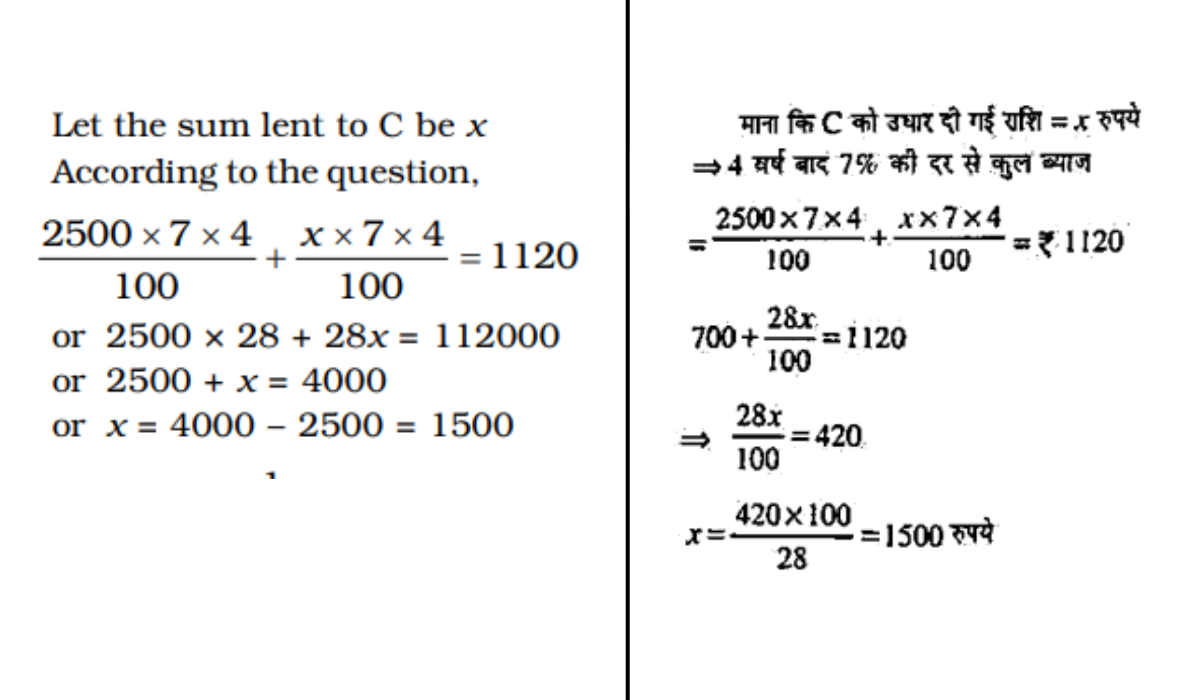• Free Test Series, Mock tests and Practice Tests
• Time proven exam strategies
• Exam analysis and simulated tests
• Hand-on real time test experience## Recently Added Articles View More >>

NEW

### Simple Interest Questions and Answers

Welcome to our Simple Interest Questions and Answers blog! If you find yourself grappling with the concepts of simple interest or looking to sharpen your skills in this fundamental area of finance, you've come to the right place.

Last month 256 Views

### Simple Interest Questions for SSC Exam

Simple Interest Questions are accessible here to assist understudies with learning the recipe and how to apply the straightforward Simple Interest equation in different issues, including genuine situations.

Last year 4.5K Views

### Simple Interest Questions and Answers - Simple Interest Formula

Simple Interest is an important topic in Mathematics. In this section, we calculate the interest on the principal amount which we borrow from any person or Bank. These types of questions are generally asked under Aptitude Section in competitive exams.

Last year 3.4K Views

### Simple Interest Questions for Competitive Exams

Simple interest is the most important part of the mathematics subject and often questions are asked in all competitive examinations. Here today in this article, we have covered simple interest-based questions to help the aspirants, which will help you to solve stressful questions in the exam and build a strong foundation.

3 years ago 8.7K Views
POPULAR

### Simple Interest and Compound Interest Problems and Solutions

In the competitive exams, there are two ways to calculate the interest, first is simple interest and second is compound interest. Both chapters are important from the perspective of SSC and Banking exams.

3 years ago 24.6K Views
POPULAR

### Problems on Simple Interest with Solutions for Competitive Exams

As you know simple interest problems are important for ssc and bank exams. Here are the some different questions with answers or solutions which asked mostly in the competitive exams. So, you should strong more your preparation by practice with these questions-answers or Problems with solutions.

3 years ago 14.1K Views
POPULAR

### Problems on Simple Interest and Compound Interest for Bank Exams

Simple interest and compounnd interest questions are mostly ask in banking exams and other competitive exams. In the simple interest questions you have to calculate interest according to the time and rate whereas in compound interest, the interest is calculated on interest.

3 years ago 16.0K Views
POPULAR

### Simple Interest Problems with Solutions for Bank Exams

Simple interest is an important topic of aptitude section. If you are preparing for banking exams, then here are simple interest problems with solutions are given and very helpful for you. In this topic, you have to calculate interest according to the time and rate.

3 years ago 15.2K Views

• 1
700
Correct
Wrong
• 2
6500
Correct
Wrong
• 3
4000
Correct
Wrong
• 4
1500
Correct
Wrong
• Workspace

## Answer : 4 1500 Explanation :• 1
Rs. 96000
Correct
Wrong
• 2
Rs. 80000
Correct
Wrong
• 3
Rs. 48000
Correct
Wrong
• 4
Rs. 60000
Correct
Wrong
• 5
None of these
Correct
Wrong
• Workspace

• 1
6: 3: 2
Correct
Wrong
• 2
2: 3: 4
Correct
Wrong
• 3
3: 4: 6
Correct
Wrong
• 4
3: 4: 2
Correct
Wrong
• Workspace

• 1
20 years
Correct
Wrong
• 2
30 years
Correct
Wrong
• 3
10 years
Correct
Wrong
• 4
22 years
Correct
Wrong
• Workspace

## Answer : 1 20 years

• 1
6.06%
Correct
Wrong
• 2
6.07%
Correct
Wrong
• 3
6.08%
Correct
Wrong
• 4
6.09%
Correct
Wrong
• Workspace

Error Reported Successfully

Error Reported Successfully